Next: Boundary Value Problems with Up: Electrostatics in Dielectric Media Previous: Polarization

# Boundary Conditions for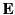and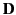If the region in the vicinity of a collection of free charges contains dielectric material of non-uniform dielectric constant then the electric field no longer has the same form as in a vacuum. Suppose, for example, that space is occupied by two dielectric media whose uniform dielectric constants are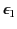and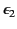. What are the matching conditions onandat the interface between the two media?

Imagine a Gaussian pill-box enclosing part of the interface. The thickness of the pill-box is allowed to tend towards zero, so that the only contribution to the outward flux ofcomes from its two flat faces. These faces are parallel to the interface, and lie in each of the two media. Their outward normals are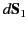(in medium 1) and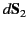, where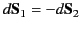. Assuming that there is no free charge inside the pill-box (which is reasonable in the limit that the volume of the box tends towards zero), Equation (503) yields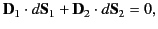(508)

where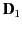is the electric displacement in medium 1 at the interface with medium 2, et cetera. The above equation can be rewritten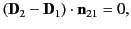(509)

where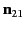is the normal to the interface, directed from medium 1 to medium 2. If the fields and charges are non-time-varying then Maxwell's equations yield(510)

which gives the familiar boundary condition (obtained by integrating around a small loop that straddles the interface)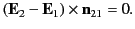(511)

In other word, the normal component of the electric displacement, and the tangential component of the electric field, are both continuous across any interface between two dielectric media.Next: Boundary Value Problems with Up: Electrostatics in Dielectric Media Previous: Polarization
Richard Fitzpatrick 2014-06-27